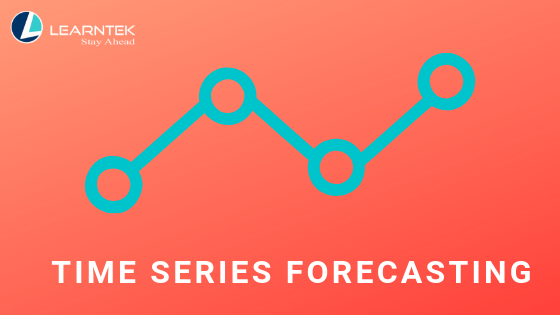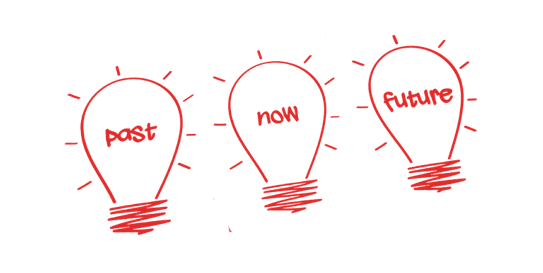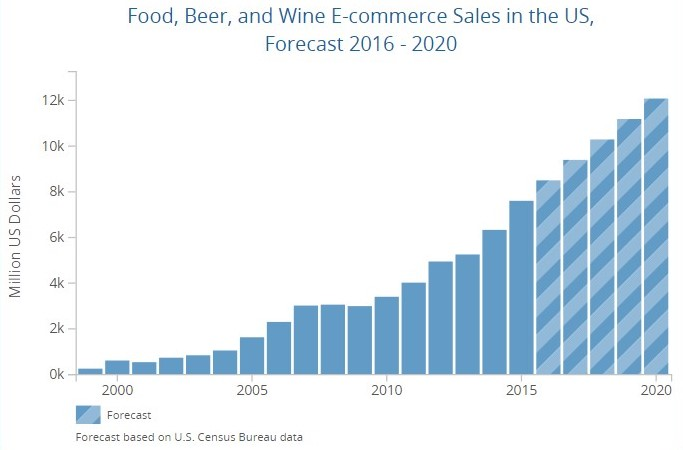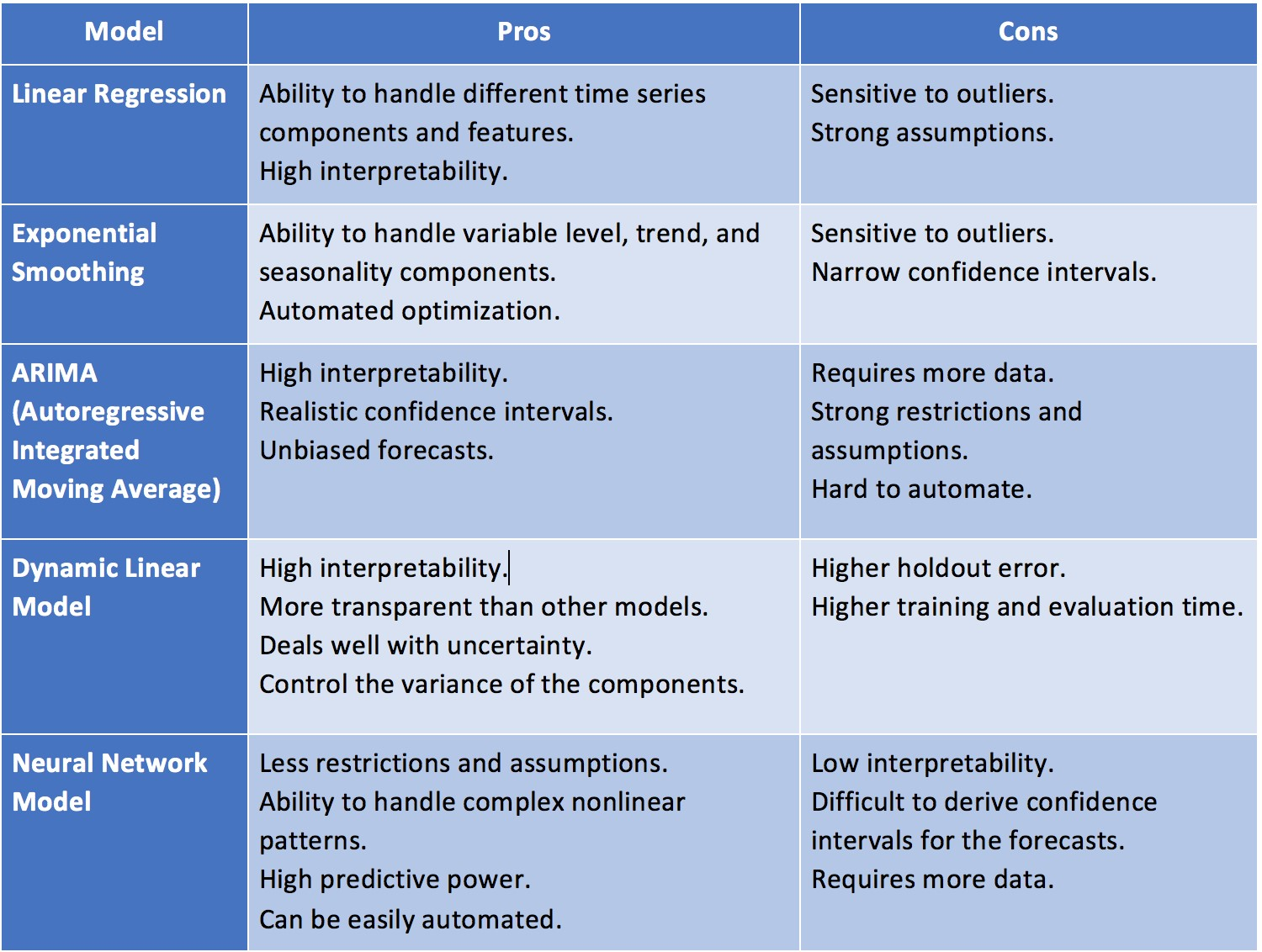# Time Series Forecasting

Time Series Forecasting is an important area of Machine Learning that is often Neglected. Time Series Forecasting uses different Technologies like Machine learning, Artificial neural networks, Support vector machines, Fuzzy logic, Gaussian processes, Hidden Markov models

What is Time Series?

A time series is a sequence of measurements done over time, usually obtained at equally spaced intervals, be it daily, monthly, quarterly or yearly. Time series analysis comprises methods for analyzing time series data in order to extract meaningful statistics and other characteristics of the data. Time series forecasting is the use of a model to predict future values based on previously observed values.

In other words, a time series is a sequence of data points being recorded at specific times.

Some of the examples of time series may be:

• Daily air temperature or monthly precipitation in Bangalore, India
• Annual flow volume of the River Ganga at Patna
• Annual Indian population data
• Daily closing stock prices
• Weekly interest rates

What is Forecasting?

Forecasting is the process of making predictions of the future based on past and present data along with analyzing the trends. Forecasting involves taking models that fit on historical data and using them to predict future observations.Time Series Analysis Techniques:

Time Series can be defined as an ordered sequence of values of a variable at equally spaced time intervals. The motivation to study time series models is twofold:

• Obtain an understanding of the underlying forces and structure that produced the observed data
• Fit a model and proceed to forecasting, monitoring or even feedback and feedforward control

Time Series Analysis can be divided into two main categories depending on the type of the model that can be fitted. The two categories are:

• Kinetic Model: The data here is fitted as xt= f(t). The measurements or observations are seen as a function of time.
• Dynamic Model: The data here is fitted as xt= f(xt-1 , xt-2 , xt-3 … ).

Machine Learning Certification Training

Time Series Forecasting Methods:

Time series forecasting methods produce forecasts based solely on historical values and they are widely used in business situations where forecasts of a year or less are required. These methods used are particularly suited to Sales, Marketing, Finance, Production planning etc. and they have the advantage of relative simplicity. Time series forecasting is a technique for the prediction of events through a sequence of time.

The technique is used across many fields of study, from geology to economics. The techniques predict future events by analyzing the trends of the past, on the assumption that the future trends will hold similar to historical trends. Data is organized around relatively deterministic timestamps, and therefore, compared to random samples, may contain additional information that is tried to extract.

• Time series methods are better suited for short-term forecasts (i.e., less than a year).
• Time series forecasting relies on sufficient past data being available and that the data is of a high quality and truly representative.
• Time series methods are best suited to relatively stable situations. Where substantial fluctuations are common and underlying conditions are subject to extreme change, then time series methods may give relatively poor results.

Examples may include:

• Forecasting the potato yield in tons by state each year
• Forecasting unemployment for a state each quarter
• Forecasting the birth rate at all hospitals in a city each yearThe plot below depicts the food, beer and wine sales in the U.S. for the year 2016 till 2020.

Basic Steps of Time Series Forecasting:

A  Time Series Forecasting task usually involves five basic steps.

Step 1: Problem definition.

Step 2: Gathering information.

Step 3: Preliminary (exploratory) analysis.

Step 4: Choosing and fitting models.

Step 5: Using and evaluating a forecasting model.

There are many statistical techniques available for time series forecast however we have found few effectives ones which are listed below:

• Simple Moving Average (SMA)
• Exponential Smoothing (SES)
• Autoregressive Integration Moving Average (ARIMA)
• Neural Network (NN)
• Croston

Some of the other Time-series forecasting methods are:

Trend Projection: This method used the underlying long-term trend of time series of data to forecast its future values.

Trend and Seasonal Components Method: This method uses seasonal component of a time series in addition to the trend component.

Causal Method: This method uses the cause-and-effect relationship between the variable whose future values are being forecasted and other related variables or factors. The widely known causal method is called regression analysis, a statistical technique used to develop a mathematical model showing how a set of variables is related. This mathematical relationship can be used to generate forecasts. There are more complex time-series techniques as well, such as ARIMA and Box-Jenkins models.

In many modern applications, time series forecasting uses computer technologies, including:

• Machine learning
• Artificial neural networks
• Support vector machines
• Fuzzy logic
• Gaussian processes
• Hidden Markov models

Concerns of Time Series Forecasting:

How much data is available and how much data you are able to gather? More data is often more helpful, offering greater opportunity for exploratory data analysis.

What is the time horizon of predictions that is required? Shorter time horizons are often easier to predict with higher confidence.

Can forecasts be updated frequently over time? Updating forecasts results in more accurate predictions.

Time series data often requires pre and post processing including cleaning, scaling, and even transformation.

Models used for Time series Forecasting:Applications of Time Series Forecasting:

Time series Forecasting models are used in :

• Finance to forecast stock’s performance
• Finance to forecast interest rate
• It is used in forecasting weather
• It is used in Budget Analysis
• It is used in Military planning
• It is used in Workload projections